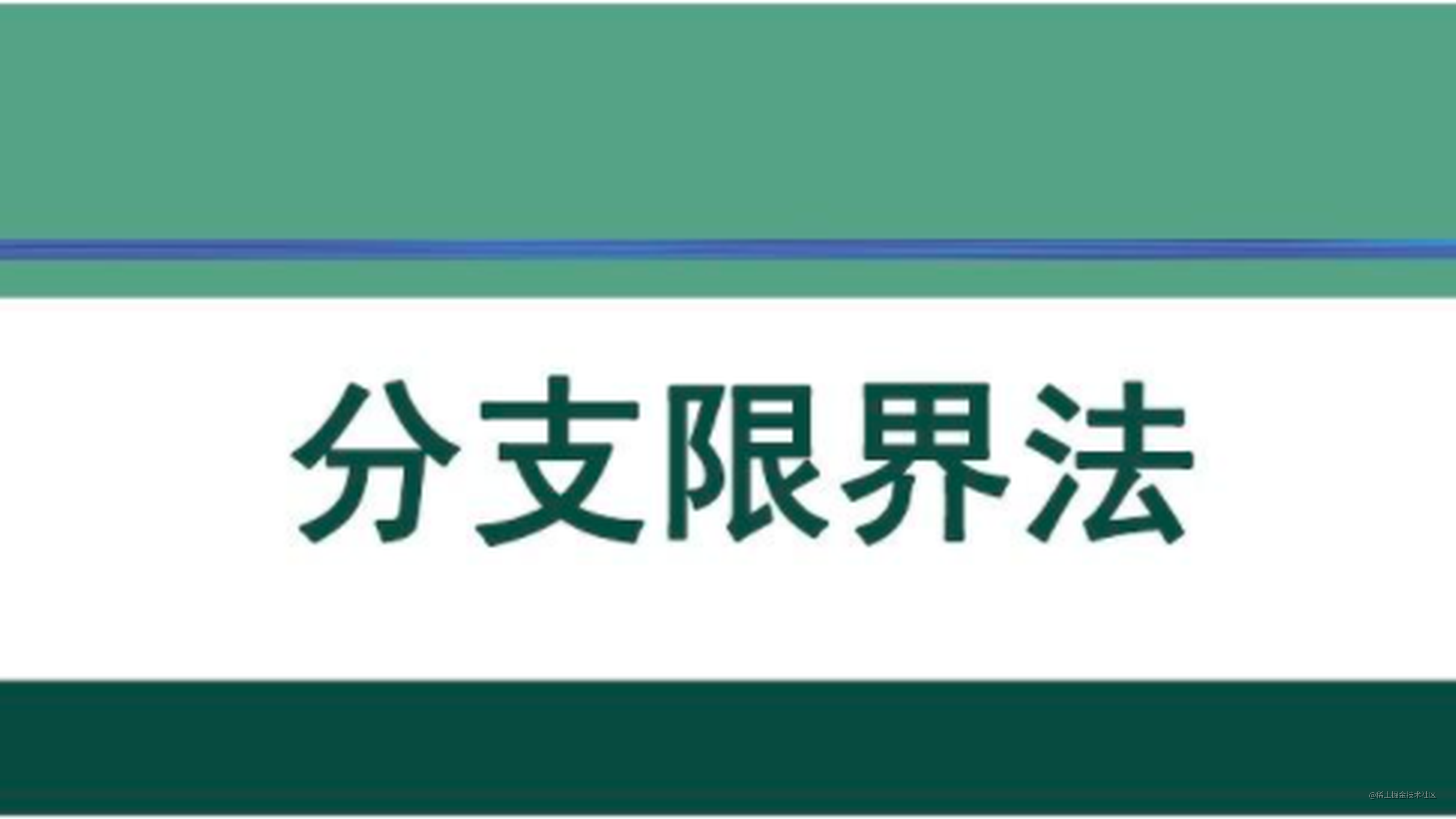# 数据结构与算法 ｜分支限界法• FIFO 搜索
• LIFO 搜索
• 优先队列式搜索

# 案例分析

**物品 **重量（w）价值（v）价值/重量（v/w）
1881
216140.875
321160.762
417110.64
51270.583

## 代码实现

``````package cn.zhengsh.t;

import java.util.PriorityQueue;

//定义节点中的参数以及优先级设置的对象
class ThingNode implements Comparable<ThingNode> {
int weight;//该节点目前背包中的重量
double value;//该节点目前背包中的总价值
double upProfit;//该节点能够达到的价值上界
int left;    //该节点是否属于左节点（用于最终构造最优解）
int level;  //该节点是第几个物品的选择
ThingNode father; //该节点的父节点

public int compareTo(ThingNode node) {
return Double.compare(node.upProfit, this.upProfit);
}

public ThingNode() {
}

public ThingNode(int weight, double value, double upProfit, int left, int level, ThingNode father) {
this.weight = weight;
this.value = value;
this.upProfit = upProfit;
this.left = left;
this.level = level;
this.father = father;
}
}

public class Bag01 {

int n = 5;
int capacity = 37;
// 已经按照价值排序排序
int[] weight = {8, 16, 21, 17, 12};
double[] value = {8, 14, 16, 11, 7};
int maxValue = 0;
int[] bestWay = new int[n];

public void getMaxValue() {
PriorityQueue<ThingNode> pq = new PriorityQueue<>();
//构造一个初始化节点，属于-1层
ThingNode initial = new ThingNode();
initial.level = -1;
initial.upProfit = 26;
while (!pq.isEmpty()) {
ThingNode fatherNode = pq.poll();
//当已经搜索到叶子节点时
if (fatherNode.level == n - 1) {
if (fatherNode.value > maxValue) {
maxValue = (int) fatherNode.value;
for (int i = n - 1; i >= 0; i--) {
bestWay[i] = fatherNode.left;
fatherNode = fatherNode.father;
}
}
} else {
//先统计其左节点信息，判断是否加入队列。
if (weight[fatherNode.level + 1] + fatherNode.weight <= capacity) {
ThingNode newNode = new ThingNode();
newNode.level = fatherNode.level + 1;
newNode.value = fatherNode.value + value[fatherNode.level + 1];
newNode.weight = weight[fatherNode.level + 1] + fatherNode.weight;
newNode.upProfit = bound(newNode);
newNode.father = fatherNode;
newNode.left = 1;
if (newNode.upProfit > maxValue)
}
//向右节点搜索，其能够取到的价值上界通过父亲节点的上界减去本层物品的价值。
if ((fatherNode.upProfit - value[fatherNode.level + 1]) > maxValue) {
ThingNode newNode2 = new ThingNode();
newNode2.level = fatherNode.level + 1;
newNode2.value = fatherNode.value;
newNode2.weight = fatherNode.weight;
newNode2.father = fatherNode;
newNode2.upProfit = fatherNode.upProfit - value[fatherNode.level + 1];
newNode2.left = 0;
}

}
}
}

//用于计算该节点的最高价值上界
public double bound(ThingNode node) {
double maxLeft = node.value;
int leftWeight = capacity - node.weight;
int templevel = node.level;
//尽力依照单位重量价值次序装剩余的物品
while (templevel <= n - 1 && leftWeight > weight[templevel]) {
leftWeight -= weight[templevel];
maxLeft += value[templevel];
templevel++;
}
//不能装时，用下一个物品的单位重量价值折算到剩余空间。
if (templevel <= n - 1) {
maxLeft += value[templevel] / weight[templevel] * leftWeight;
}
return maxLeft;
}

public static void main(String[] args) {
Bag01 b = new Bag01();
b.getMaxValue();
System.out.println("该背包能够取到的最大价值为:" + b.maxValue);
System.out.println("取出的方法为:");
for (int i : b.bestWay)
System.out.print(i + "  ");
}
}

1. 0/1背包问题
2. 单源最短路径问题
3. 最优装载问题
4. 单源最短路径问题
5. 最大团问题
6. 旅行商问题
7. 电路板排列问题